# Energy and charge stored in a capacitor

The energy and charge stored in a capacitor can be calculated if the voltage and capacitance are known.

### Formulas

The following formulas can be used to calculate the energy and charge in a capacitor. You can also calculate the voltage or capacitance if the other values are known.

#### Energy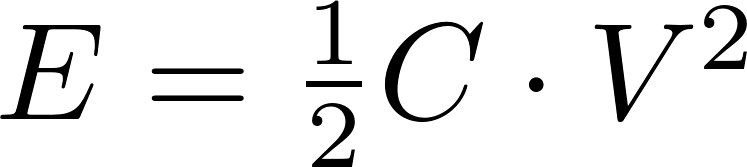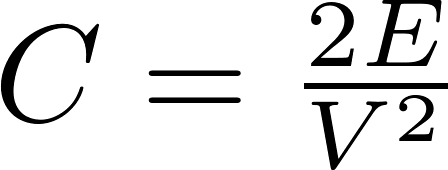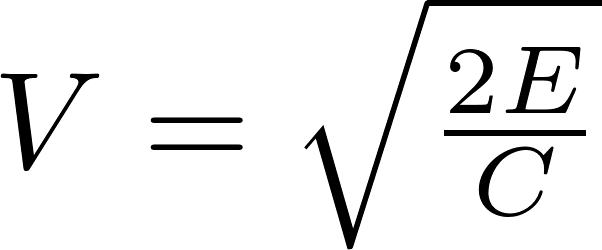#### Charge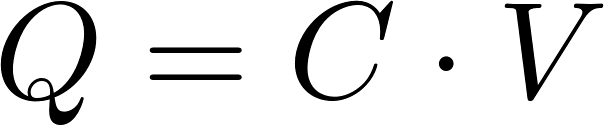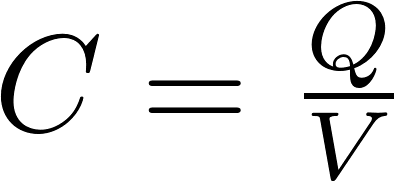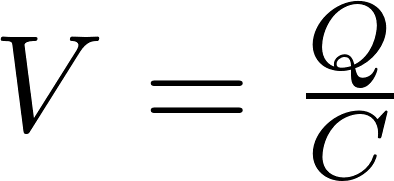The following formula is needed if you want to calculate the capacitance when only the stored energy and charge are known: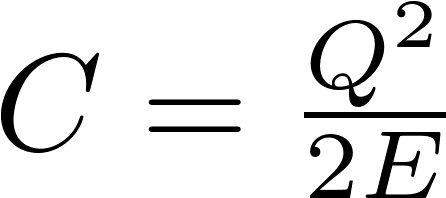E is the symbol for energy and is measured in joule (J).
C is the symbol for capacitance and is measured in farad (F).
V is the symbol for voltage and is measured in volt (V).
Q is the symbol for charge and is measured in coulomb (C).